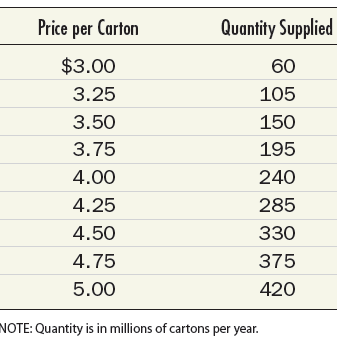# Now suppose the supply schedule is instead as follows: What are the equilibrium price and equilibrium quantity in the absence of a tax? What are the equilibrium price and equilibrium quantity in the presence of a \$1.25 per carton excise tax? Explain why your answer to part b differs from your answer to part b of the previous question, and relate this difference to the discussion of the incidence of an excise tax in this chapter.### Microeconomics: Principles & Policy

14th Edition
William J. Baumol + 2 others
Publisher: Cengage Learning
ISBN: 9781337794992

#### Solutions

Chapter
Section### Microeconomics: Principles & Policy

14th Edition
William J. Baumol + 2 others
Publisher: Cengage Learning
ISBN: 9781337794992
Chapter 17, Problem 4TY
Textbook Problem
1 views

## Now suppose the supply schedule is instead as follows:What are the equilibrium price and equilibrium quantity in the absence of a tax? What are the equilibrium price and equilibrium quantity in the presence of a \$1.25 per carton excise tax? Explain why your answer to part b differs from your answer to part b of the previous question, and relate this difference to the discussion of the incidence of an excise tax in this chapter.

This textbook solution is under construction.

### Still sussing out bartleby?

Check out a sample textbook solution.

See a sample solution

#### The Solution to Your Study Problems

Bartleby provides explanations to thousands of textbook problems written by our experts, many with advanced degrees!

Get Started